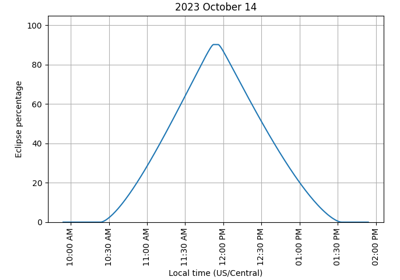# eclipse_amount#

sunpy.coordinates.sun.eclipse_amount(observer, *, moon_radius: Literal['IAU', 'minimum'] = 'IAU')[source]#

Return the percentage of the Sun that is eclipsed by the Moon.

The occultation of the solar disk by the Moon is calculated using the simplifying assumption that the Moon has a constant radius. Since the Moon has an irregular profile of peaks and valleys, the output can be slightly inaccurate for the start/end of partial solar eclipses (a.k.a. penumbral contacts) and the start/end of total solar eclipses (a.k.a. umbral contacts).

Parameters:
• observer (SkyCoord) – The observer location and observation time.

• moon_radius (str) – The choice of which radius to use for the Moon. The default option ('IAU') is the IAU mean radius (R_moon / R_earth = 0.2725076). The alternative option ('minimum') is the mean minimum radius (R_moon / R_earth = 0.272281).

Notes

The apparent location of the Moon accounts for the effect of light travel time.

The location of the Moon is appreciably inaccurate with Astropy’s built-in ephemeris, so it is highly recommended to use a JPL ephemeris instead. See Solar System Ephemerides.

Using the mean minimum radius for the Moon will result in slightly more accurate estimates of the start/end of total solar eclipses, at the expense of slightly more inaccurate estimates for the amount of partial solar eclipses. See this page for relevant discussion.

ExamplesObtaining solar-eclipse information

Obtaining solar-eclipse information